# Extract Last Two Words From Multiple Cells

Just assume that you have a few cells containing values/words and you want to extract the last two words from each cell into another separate cell; then you might think that it’s not a big deal; because you would prefer to manually extract the last two words from the cell into another without any need of the formula then congratulations because you are thinking right, but let me add up that it would be a big deal to extract the last two words from the multiple cells to another cell and doing it manually would be a foolish attempt because you would get tired of it and would never complete your work on time.

But don’t be worry about it because after carefully reading this article, extracting last the two words from multiple cells into separate cells would become a piece of cake for you.

So let’s dive into the article to take you out of this fix.

## General formula:

The Following formula would help you out for extracting last the two words from multiple cells into separate cells :

`=MID(B1,FIND("#",SUBSTITUTE(B1," ","#",LEN(B1)-LEN(SUBSTITUTE(B1," ",""))-1))+1,200)`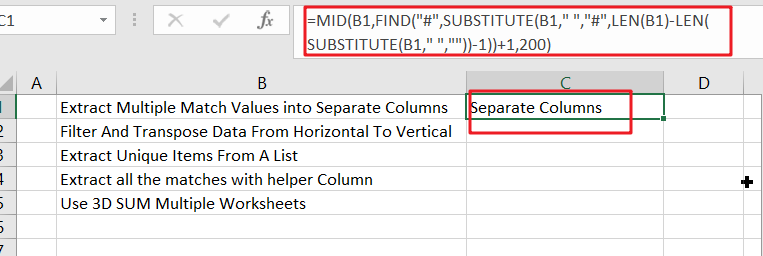## Syntax Explanations:

Before going into the explanation of the formula for getting the work done efficiently, we must understand each syntax which would make it easy for you that how each syntax contributes to extracting the last two words/values from multiple cells into separate cells:

• `MID`: This function contributes to extracting the number or characters from the given string by starting from the left side.
• `FIND`: In Excel, this FIND function contributes to finding out one text inside the other one.
• `LEN`: In Excel, this LEN function contributes to finding out the length of the text string.
• `SUBSTITUTE`: In excel, this SUBSTITUTE function contributes to replacing the existing text with new text in a text string.
• B1: this function represents the input value.
• Comma symbol (,): In Excel, this comma symbol acts as a separator that helps to separate a list of values.
• Parenthesis (): The core purpose of this Parenthesis symbol is to group the elements and to separate them from the rest of the elements.
• Minus Operator (-): This minus symbol contributes to subtracting any two values.
• Plus operator (+): This plus symbol adds the values.

## Let’s See How This Formula Works:

The formula uses the MID function to extract the characters from the second to last space. The MID function accepts three arguments; the number of characters to extract, the starting position, the text to work with.

The text is from column B, and the number of characters can be large enough to ensure that the last two words are taken. The task is to figure out where to start, just after the second-to-last spot. The sophisticated work is mostly done with the SUBSTITUTE function, which accepts an optional input called instance number. This function is used to replace the second to last space in the text with the “#” character, which is then found using the FIND function.

The following snippet figures out how many total spaces are in the text, from which 1 is subtracted.

`=LEN(B1)-LEN(SUBSTITUTE(B1," ","")-1`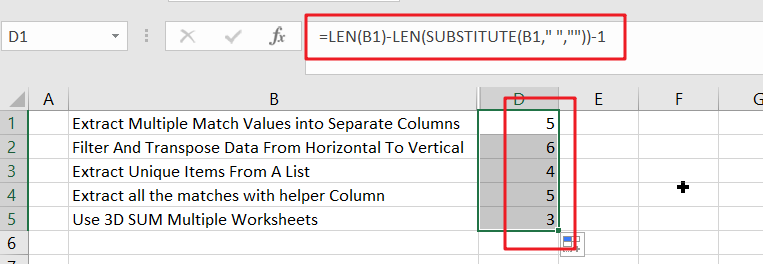According to the example, the above code would return 5 because there are 6 spaces in the text. As the instance number, this returning number is then placed into the SUBSTITUTE function.

`=SUBSTITUTE(B1," ","#",5)`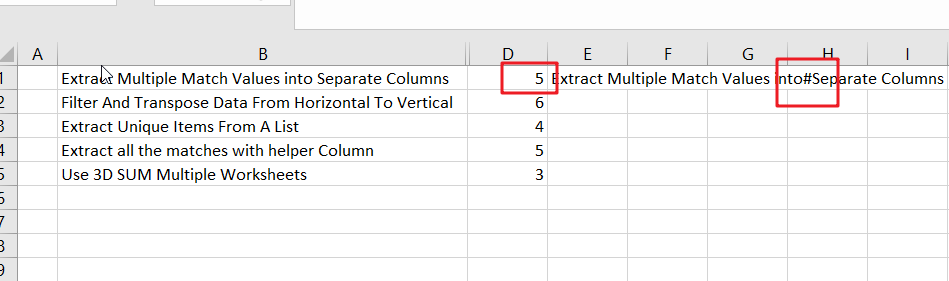Due to the above placement of the returning number, the SUBSTITUTE function would replace the fifth space character with “`#`“, now you might be curious about it that why we are using “`#`” ? so here is the answer that it’s an arbitrary choice you can use any other character too but here is the condition that the chosen character would not appear in the original text.

After this, the FIND Function would locate the “`#`” character (or whatever character you would use) in the text:

`=FIND("#","Extract Multiple Match Values into#Separate Columns")`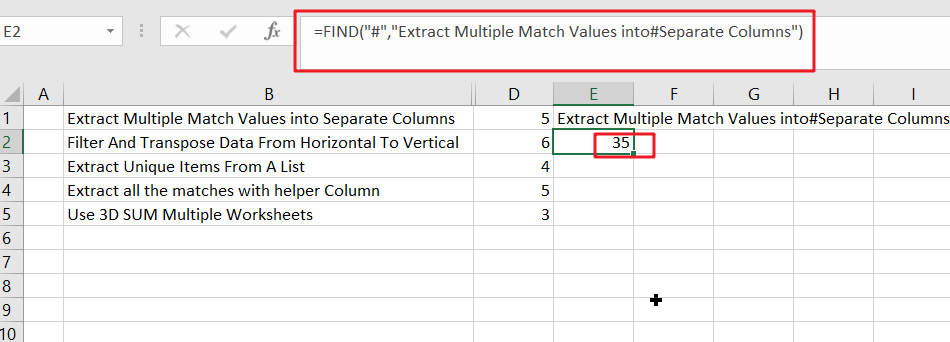According to the example, the result of the FIND function would be 35, in which 1 is added for getting 36. This is the starting point, and as the second argument, it would go into the MID function.

## Extract Last N words from String Using MID Function

In this formula, we have made the adjustments to extract the last 2 words from the cells and can also be generalized to extract the last N words from a cell by replacing the hardcoded 1 in the example with (N-1).

Moreover, if you want to extract many words, you must replace the hardcoded argument in MID, 200, with a larger number. To make sure that the number is large enough, you can use the LEN function, like it is used as follows:

=MID(B1,FIND(“#”,SUBSTITUTE(B1,” “,”#”,LEN(B1)-LEN(SUBSTITUTE(B1,” “,””))-1))+1,LEN(B1))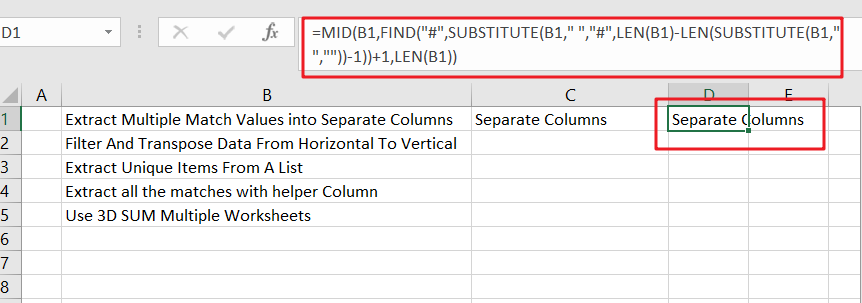### Related Functions

• Excel MID function
The Excel MID function returns a substring from a text string at the position that you specify.The syntax of the MID function is as below:= MID (text, start_num, num_chars)…
• Excel Substitute function
The Excel SUBSTITUTE function replaces a new text string for an old text string in a text string. The syntax of the SUBSTITUTE function is as below:= SUBSTITUTE  (text, old_text, new_text,[instance_num])….
• Excel LEN function
The Excel LEN function returns the length of a text string (the number of characters in a text string).The LEN function is a build-in function in Microsoft Excel and it is categorized as a Text Function.The syntax of the LEN function is as below:= LEN(text)…
• Excel FIND function
The Excel FIND function returns the position of the first text string (sub string) within another text string.The syntax of the FIND function is as below:= FIND(find_text, within_text,[start_num])
Related Posts

Find and Replace Multiple Values

This post will guide you how to find and replace multiple values at once with VBA macro or using formula  in Excel. How do I make multiple find and replace in Excel. Suppose that you have a few cells containing ...

Extract Multiple Lines From A Cell

Suppose that you have listed some text in a single cell which is separated by the line break(you can do it by pressing ALT + ENTER after entering the text), and now you want to extract multiple lines of text ...

How to Remove All Asterisk Characters from Cells in Excel

Sometimes when we opening an excel spreadsheet to check data, we may notice that there are some asterisks exist in texts for security or other reasons. If you feel these special characters are invalid you can remove them from cells. ...

How to Convert Date Format from Dot MM.DD.YYYY to Slash MM/DD/YYYY in Excel

We often enter dates in excel tables, and we can enter current date by quickly press ‘Ctrl+;’ simultaneously. If in some cases we enter a date format that is not what we want, we have to switch the date format ...

How to Remove Quotes for Text or Strings in Excel

When we importing data from other source into Excel we may import quotes or quotations from initial file. And we want to remove these quotes from current file, if we remove them one by one it needs a lot of ...

How to Remove Specific Character from Text Cell in Excel

This post will guide you how to remove a specific character from text cell in Excel. How do I remove certain character from a cell with a formula in Excel 2013/2016. Remove Specific Character Using Find and Replace function Remove ...

How to Count Comma Separated Value in One Cell in Excel

This post will guide you how to count values in a single cell separated by commas with a formula in Excel. How do I count comma separated values in one cell in Excel 2013/2016. Is it possible to have a ...

How to Count the Number of Letters or Numbers separately in a Cell in Excel

This post will guide you how to count the number of a given character in a cell in Excel. How do I count only numbers within a single cell excluding all letters and other characters with a formula in Excel. ...

How to Remove the First/Last Word from Text string in Cell

This post will guide you how to remove the first and the last word from a text string in cells in Excel. How do I use a formula to remove first and last word of a text string in Excel. ...

How to Remove Line Breaks (Carriage Returns) in Excel

This post will explain that how to remove line breaks in your data set in Excel. How do I remove carriage returns or line breaks with a formula in Excel. How to remove line breaks with VBA Macro in Excel. ...

Sidebar